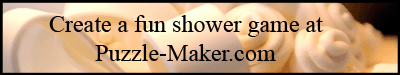Why all the W's?

R  L  T  S  R  O  W  W  H  I  M  W  Y

S  L  D  C  E  T  O  R  W  Q  I  E  E

U  E  P  N  O  W  N  A  W  L  W  R  E

W  W  J  T  A  W  E  P  L  H  E  W  A

H  W  B  W  I  M  W  R  S  W  S  A  A

K  S  A  N  O  P  O  O  H  N  T  S  W

D  U  I  L  N  K  O  W  V  S  E  O  H

Z  L  X  W  L  H  E  W  W  E  S  H  Y

U  P  U  R  W  W  E  A  G  K  N  W  W

W  U  T  O  I  B  K  I  Y  R  O  M  X

A  Y  E  T  W  E  W  W  W  W  I  C  K

Y  X  W  H  E  Y  Z  A  Y  N  O  B  T

F  W  A  D  G  G  T  R  G  A  W  D  N

 Wad Were Win Wag West Wish Wake Wet Wit Wall When Woke Wan Whey Woman War Whim Won Was Whoosh Worst Way Why Would Web Wick Woven Wed Wig Wow Well Will Wrote